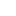v2.25IGrid Interface Reference

Represents a triangle grid. More...

Inheritance diagram for IGrid:## Public Member Functions

HRESULT GetTriangle ([in] int index, [out, retval] Triangle *pTriangle)
Returns the triangle by the given index. More...

HRESULT GetVertex ([in] int index, [out, retval] FloatPoint3D *pPoint)
Returns the vertex by the given index. More...

HRESULT GetNormal ([in] int index, [out, retval] FloatVector3D *pVector)
Returns the normal vector by the given index. More...

HRESULT GetTextureCoordinate ([in] int index, [out, retval] FloatPoint2D *pPoint)
Returns the texture coordinates by the given index. More...

HRESULT GetTriangleComponents ([in] int index, [out] unsigned int *pTriangleV0, [out] unsigned int *pTriangleV1, [out] unsigned int *pTriangleV2)
Returns the triangle components by the given index. More...

HRESULT GetVertexComponents ([in] int index, [out] float *pX, [out] float *pY, [out] float *pZ)
Returns the vertex components by the given index. More...

HRESULT GetNormalComponents ([in] int index, [out] float *pX, [out] float *pY, [out] float *pZ)
Returns the normal vector components by the given index. More...

HRESULT GetTextureCoordinateComponents ([in] int index, [out] float *pX, [out] float *pY)
Returns the texture coordinates components by the given index. More...

## Properties

int TriangleCount` [get]`
The number of triangles in the grid. More...

int VertexCount` [get]`
The number of vertices in the grid. More...

int NormalCount` [get]`
The number of normals in the grid. More...

int TextureCoordinateCount` [get]`
The number of texture coordinates in the grid. More...

int GridType` [get]`
The grid type. More...

VARIANT_BOOL DoubleSided` [get]`
Queries if the grid is double-sided. More...

## Detailed Description

Represents a triangle grid.

A grid is a subset of a mesh corresponding to a particular face of an exported object. This interface provides access to grid vertices and their normals and texture coordinates.

Most often, vertex, normal and texture coordinate vector counts are equal. It means that any vertex has a corresponding normal vector and a texture coordinate vector. But in some cases it is possible that a vertex has no normal vector and/or texture coordinate. (One such case when it might happen is when objects are imported into Renga from another CAD.) Therefore you should not assume the numbers of vertices, normal vectors and texture coordinates to be equal. For example:

Renga::IGridPtr grid = mesh->GetGrid(someIndex);
bool hasNormals = grid->GetNormalCount() == grid->GetVertexCount();
bool hasTexCoords = grid->GetTextureCoordinateCount() == grid->GetVertexCount();
for (int i = 0; i < grid->GetVertexCount(); i++)
{
Renga::Point3D vertex = grid->GetVertex(i);
if (hasNormals && hasTexCoords)
{
Renga::Vector3D normal = grid->GetNormal(i);
Renga::Point2D texCoord = grid->GetTextureCoordinate(i);
// do something with vertex, normal, texCoord
}
else
{
// do something with vertex only
}
}
IMesh, IExportedObject3D, Point3D, Vector3D, Triangle, gridTypes

## ◆ GetNormal()

 HRESULT IGrid::GetNormal ( [in] int index, [out, retval] FloatVector3D * pVector )

Returns the normal vector by the given index.

Parameters
 [in] index Index. [out] pVector The requested normal vector.
NormalCount

## ◆ GetNormalComponents()

 HRESULT IGrid::GetNormalComponents ( [in] int index, [out] float * pX, [out] float * pY, [out] float * pZ )

Returns the normal vector components by the given index.

Parameters
 [in] index Index. [out] pX The x component of the requested normal vector. [out] pY The y component of the requested normal vector. [out] pZ The z component of the requested normal vector.
NormalCount

## ◆ GetTextureCoordinate()

 HRESULT IGrid::GetTextureCoordinate ( [in] int index, [out, retval] FloatPoint2D * pPoint )

Returns the texture coordinates by the given index.

Parameters
 [in] index Index. [out] pPoint The requested texture coordinate.
TextureCoordinateCount

## ◆ GetTextureCoordinateComponents()

 HRESULT IGrid::GetTextureCoordinateComponents ( [in] int index, [out] float * pX, [out] float * pY )

Returns the texture coordinates components by the given index.

Parameters
 [in] index Index. [out] pX The x component of the requested texture coordinate point. [out] pY The y component of the requested texture coordinate point.
TextureCoordinateCount

## ◆ GetTriangle()

 HRESULT IGrid::GetTriangle ( [in] int index, [out, retval] Triangle * pTriangle )

Returns the triangle by the given index.

Parameters
 [in] index Index. [out] pTriangle The requested triangle.
TriangleCount

## ◆ GetTriangleComponents()

 HRESULT IGrid::GetTriangleComponents ( [in] int index, [out] unsigned int * pTriangleV0, [out] unsigned int * pTriangleV1, [out] unsigned int * pTriangleV2 )

Returns the triangle components by the given index.

Parameters
 [in] index Index. [out] pTriangleV0 The first vertex index of the requested triangle. [out] pTriangleV1 The second vertex index of the requested triangle. [out] pTriangleV2 The third vertex index of the requested triangle.
TriangleCount

## ◆ GetVertex()

 HRESULT IGrid::GetVertex ( [in] int index, [out, retval] FloatPoint3D * pPoint )

Returns the vertex by the given index.

Parameters
 [in] index Vertex index in the collection. [out] pPoint The requested point with coordinates of the vertex.
VertexCount

## ◆ GetVertexComponents()

 HRESULT IGrid::GetVertexComponents ( [in] int index, [out] float * pX, [out] float * pY, [out] float * pZ )

Returns the vertex components by the given index.

Parameters
 [in] index Vertex index in the collection. [out] pX The x component of the requested point with coordinates of the vertex. [out] pY The y component of the requested point with coordinates of the vertex. [out] pZ The z component of the requested point with coordinates of the vertex.
VertexCount

## ◆ DoubleSided

 VARIANT_BOOL IGrid::DoubleSided
get

Queries if the grid is double-sided.

Orientation of the grid sides (i.e. which side is the inner one, and which is the outer) is the same as the orientation of the ExportedObject3D face it represents.

## ◆ GridType

 int IGrid::GridType
get

The grid type.

Grid types

## ◆ NormalCount

 int IGrid::NormalCount
get

The number of normals in the grid.

GetNormal()

## ◆ TextureCoordinateCount

 int IGrid::TextureCoordinateCount
get

The number of texture coordinates in the grid.

GetTextureCoordinate()

## ◆ TriangleCount

 int IGrid::TriangleCount
get

The number of triangles in the grid.